# Alternatives to LibreOffice - Math

• ### fxSolver

Online math solver, equation library, graphing calculator and science/engineering problem helper.Calculate multiple equations at once, Edit existing formulas and Create new ones, Compute large numbers of values, Plot graphs, Link your results, Solve…

Free Web• ### MathType

MathType is a powerful interactive equation editor for Windows and Macintosh that lets you create mathematical notation for word processing, web pages, desktop publishing, presentations, elearning, and for TeX, LaTeX, and MathML documents. Discount …

Commercial Windows Mac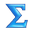• ### KLatexFormula

KLatexFormula is an easy-to-use graphical application for generating images (that you can drag and drop, copy and paste or save to disk) from LaTeX equations. It is written in Qt4. These images can be dragged and dropped or copied and pasted into ex…

Open Source Free Linux Windows Mac• ### LaTeXiT

LaTeXiT is a small utility that allows you to quickly typeset LaTeX equations, without bothering with file creation, preambles, and so on. The PDF image obtained can then be exported by dragn drop to any application supporting it. This is very usefu…

Free Mac• ### MathCast

MathCast is an equation editor, an application that allows you to input mathematical equations. These equations can be used in written documents, webpages, and even databases. They could be rendered graphically to the screen, to picture files, or to…

Open Source Free Windows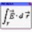• ### Apache OpenOffice Math

Math, part of the Apache OpenOffice suite, is a tool for creating and editing mathematical equations, similar to Microsoft Equation Editor (included in Microsoft Office). The created equations can then be embedded inside other OpenOffice.org documen…

Open Source Free Linux Windows Mac• ### EqualX

EqualX Equation Editor - is a powerful interactive tool that lets you create mathematical notation for word processing, web pages, desktop publishing, presentations in TeX, LaTeX

Open Source Free Linux Windows• ### Math Magic

MathMagic Equation Editor for Desktop Publishing software &amp; Word Processors, Adobe InDesign, QuarkXPress, MS Word, iWork, for Technical writing with very easy interface and fast input.

Commercial Windows Mac• ### Sequalator

Sequalator stands for Simultaneous Equations Calculator.It is a software for solving Linear Simultaneous Equations. The solver is based on the Gauss Jordan elimination method for solving Linear Simultaneous Equations. Sequalator uses OpenCL technolo…

Free Windows• ### WIRIS editor

WIRIS editor is a visual editor (WYSIWYG) that allows inserting mathematical formulas in web pages. It runs on any browser, including the ones in tablet PCs, as is based on HTML4 and JavaScript technology. Plus it is of course 100% compliant with HT…

Free Web• ### Tex2Img

Tex2Img is a free online Latex equation editor that converts Latex equations to high resolution images to embed in documents and presentations. You can also use Tex2Img to learn Latex by trial and error. You can change the equation background color,…

Free Web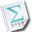• ### Ekee

Small GUI to display LaTeX expressions and then copy to clipboard or save the result. Ekee produces images interactively from LaTeX expressions. Drag and drop in several common formats (PNG, SVG, PDF, EPS) to other applications is supported. Ekee ca…

Open Source Free Linux

• ### Math Minion

Math Minion started out as a powerful scientific calculator and modelling system with a deep understanding of conversion units, but over time it has evolved into much more. When string handling was introduced to its powerful array handling, it becam…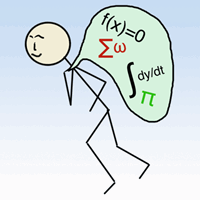• ### Visual Math Editor

VisualMathEditor is a LaTeX, AsciiMath and MathML editor developed in Javascript and HTML. It operates independently on the web or locally. It has many features and menus that help the equation-entry and allow their posting and backup.

Open Source Free Self-Hosted Web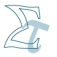• ### Equation Illustrator V

Equation Illustrator is a tool for math and technical graphic work that helps you format and align formulae.

Commercial Windows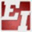• ### iMathEQ Math Equation Editor

iMathEQ is a mathematics equation or formula editor. It is a WYSIWYG online equation/formula editor. It allows users to input, edit, print math equation or expressions. Provide interface for easy integration with web site or mobile app at affordable…

Commercial Web